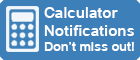Calculator Library
Free Online CalculatorsHome » 87 Calculators » Blog » How To Plot Points On A Graphing Calculator?

Huge Domain Name Sale | Free Domains
Domain names on sale at crazy prices. Register a new domain for FREE when you buy another service.

## HOW TO PLOT POINTS ON A GRAPHING CALCULATOR?When it comes to graphing calculators, many students feel overwhelmed. It can be difficult to know how to use all of the features on these devices. In this article, we'll show you how to plot points on a graphing calculator. This is an essential skill that every user should know!

## What Is Graphing?

Graphing is the process of plotting data points on a graph. This can be done by hand or with a graphing calculator. Graphing is a useful way to visualize data and see relationships between variables.

## What Is A Graphing Calculator?

A graphing calculator is a handheld device that's capable of plotting points on a graph. These calculators are often used in mathematics and science classrooms to help students visualize concepts. Graphing calculators can also be used for other purposes, such as creating graphs of data sets.

### Uses Of A Graphing Calculator

There are many uses for a graphing calculator. Some of the most common uses are:

- To graph equations: Used to graph equations. This is a useful way to visualize how an equation behaves.

- To find trends in data: Finding trends in data. This is a useful way to see how a variable changes over time.

- To make predictions: A graphing calculator can be used to make predictions. This is a useful way to extrapolate data and make estimates.

## Why Should I Learn How To Plot Points On A Graphing Calculator?Plotting points on a graphing calculator is a useful skill to have. The benefits of graphing data points on a graphing calculator are:

- You can visually see the data: When you plot points on a graphing calculator, you can visually see the data. This is helpful when you're trying to understand how a concept works.

- You can find trends in the data: When you plot points on a graphing calculator, you can find trends in the data. This is helpful when you're trying to understand how a variable changes over time.

- You can see the relationships between variables: When you plot points on a graphing calculator, you can see the relationships between variables. This is helpful when you're trying to understand how two variables interact.

- You can make predictions based on the data: When you plot points on a graphing calculator, you can make predictions based on the data. This is helpful when you're trying to extrapolate data and make estimates.

## How To Plot Points On A Graphing Calculator?

Now that we know what graphing is and why it's useful, let's learn how to plot points on a graphing calculator. The process of graphing points on a graphing calculator is relatively simple.

To graph a point on a graphing calculator, you need to enter the coordinates of the point into the calculator. The coordinates of a point are the (x, y) values of the point. For example, if you want to graph the point (0, 0), you would enter the coordinates 0, 0 into the graphing calculator.

Once you have entered the coordinates of the point, the graphing calculator will plot the point on a graph. The point will be represented by a small dot on the graph. If you want to see how the point moves, you can use the arrow keys on the graphing calculator to move the point around on the graph.

You can also enter multiple points into the graphing calculator. To do this, you need to enter the coordinates of each point, separated by a comma. For example, if you want to graph the points (0, 0) and (0, -100), you would enter the coordinates 0, 0, 0 and -100 into the graphing calculator.

The graphing calculator will plot all of the points on the graph. Each point will be represented by a small dot on the graph. If you want to see how the points move, you can use the arrow keys on the graphing calculator to move the points around on the graph.

You can also change the way the points are graphed on the calculator. To do this, you need to enter the coordinates of the point and then press the "Graph" button. The "Graph" button is located on the graphing calculator next to the "Enter" button.

Once you have pressed the "Graph" button, a menu will appear. This menu will allow you to change the way the points are graphed on the calculator. You can choose to graph the points as a line, a scatter plot or a bar graph.

### Plotting Points On TI-83/84

To plot points on a TI-83 or TI-84 graphing calculator, follow these steps:

- Press the button marked STAT.

- Enter the data points into the lists.

- Press the button marked GRAPH.

- Select the type of graph you want to create.

- Press the button marked PLOT.

- Select the type of plot you want to create.

- Enter the data points into the graphing calculator.

- Press the button marked TRACE.

- Use the arrow keys to trace the graph.

- Press the button marked Y=.

- Enter the equation you want to graph.

- Press the button marked GRAPH.

## Conclusion

Graphing points on a graphing calculator is a simple process. By entering the coordinates of the point, you can see how the point moves on the graph. You can also enter multiple points and change the way they are graphed on the calculator. Graphing points on a graphing calculator is a helpful way to visualize data.

 Previous Post« How To Find CSC On A Calculator Next PostHow To Put A Fraction On An Online Calculator? »